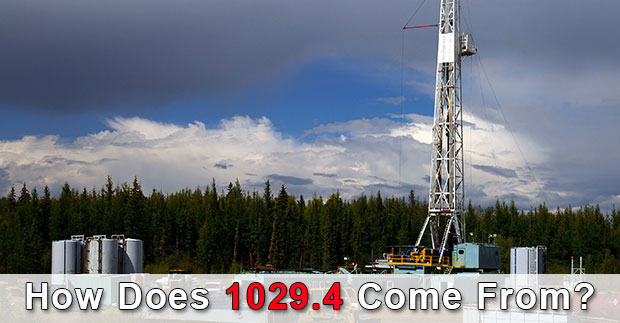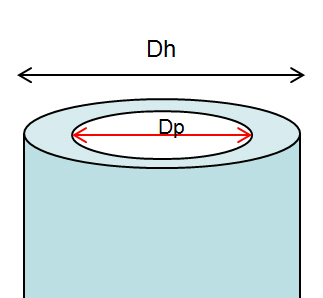## How Does 1029.4 Come From?

1029.4 is used in several calculations in the oilfield and we’ve been asked about what is 1029.4, how it comes from, why it needs to be this figure so in this article, we will show you how 1029.4 comes from.First of all, we would like to give someone about the background of this figure. The 1029.4 is widely used for capacity calculations. The following equations utilizing 1029.4 are listed below; Continue reading

## Hydrostatic Pressure (HP) Decreases When POOHWhen pulling out of hole, volume of steel will be out of hole and mud volume will replace the steel volume.  If we don’t fill hole, hydrostatic pressure will decrease.  This topic shows you how to calculate hydrostatic pressure loss while pulling out of hole without filling the wellbore.  Moreover, there is the Excel sheet for calculating pressure decrease due to pulling out of hole.

## Oilfield Unit

Step 1: Determine Total Volume of Steel Out of Hole

Total Volume of Steel Out of Hole = Length  of pipe pulled out × Pipe Displacement

Where,

Total Volume of Steel Out of Hole in bbl

Length  of pipe pulled out in ft

Pipe Displacement in bbl/ft

Step 2: Determine Hydrostatic Pressure Decrease

Hydrostatic Pressure Decrease = (Total Volume of Steel Out of Hole × 0.052 × mud weight) ÷ (casing capacity – pipe displacement)

Where,

Hydrostatic Pressure Decrease in psi

Total Volume of Steel Out of Hole in bbl

mud weight in ppg

casing capacity in bbl/ft

pipe displacement in bbl/ft

Example: Determine the hydrostatic pressure decrease when pulling pipe out of the hole:

Number of stands pulled = 10
Pipe displacement = 0.0055 bbl/ft
Average length per stand = 91 ft
Casing capacity = 0.0873 bbl/ft
Mud weight = 12.0 ppg

Step 1: Determine of pipe displacement in Barrels = 10 stands × 91 ft/std × 0.0055 bbl/ft displaced

Total Volume of Steel Out of Hole = 5.01 bbl

Step 2: Determine HP, psi decrease = 5.01 barrels × 0.052 × 12.0 ppg ÷ (0.0873 bbl/ft – 0.0055 bbl/ft)

Hydrostatic pressure decrease = 38.2 psi

## Metric Unit

Step 1: Determine Total Volume of Steel Out of Hole

Total Volume of Steel Out of Hole = Length  of pipe pulled out × Pipe Displacement

Where,

Total Volume of Steel Out of Hole in m3

Length  of pipe pulled out in m

Pipe Displacement in m3 /m

Step 2: Determine Hydrostatic Pressure Decrease

Hydrostatic Pressure Decrease = (Total Volume of Steel Out of Hole × 0.00981 × mud weight) ÷ (casing capacity – pipe displacement)

Where,

Hydrostatic Pressure Decrease in KPa

Total Volume of Steel Out of Hole in m3

mud weight in kg/m3

casing capacity in m3 /m

pipe displacement in m3 /m

Example: Determine the hydrostatic pressure decrease when pulling pipe out of the hole:

Number of stands pulled = 10
Pipe displacement = 0.00287 m3 /m
Average length per stand = 30 m
Casing capacity = 0.04554 m3 /m
Mud weight = 1440 kg/m3

Step 1: Determine of pipe displacement in m3 = 10 stands × 30 m/std × 0.00287 m3 /m pipe displacement

Total Volume of Steel Out of Hole 0.86  m3

Step 2: Determine HP, psi decrease = 0.86  m3 × 0.00981 × 1440 kg/m3 ÷ (0.04554 m3 /m- 0.00287 m3 /m)

Hydrostatic pressure decrease = 285 KPa

Please find the Excel sheet for calculating pressure decrease due to pulling out of hole.Ref books:

Lapeyrouse, N.J., 2002. Formulas and calculations for drilling, production and workover, Boston: Gulf Professional publishing.

Bourgoyne, A.J.T., Chenevert , M.E. & Millheim, K.K., 1986. SPE Textbook Series, Volume 2: Applied Drilling Engineering, Society of Petroleum Engineers.

Mitchell, R.F., Miska, S. & Aadny, B.S., 2011. Fundamentals of drilling engineering, Richardson, TX: Society of Petroleum Engineers.

## Calculate Annular CapacityAnnular capacity  is volume of fluid between two diameter of cylindrical objects per length or length per volume. This article demonstrates you how to calculate annular capacity between casing or hole and drill pipe, tubing, or casing. There are several formulas as shown below to calculate annular capacity depending on unit of annular capacity required.Note: Dh is bigger ID and Dp is smaller OD. The examples below will show the Dh as hole size and Dp is drill pipe OD## a) Calculate annular capacity in bbl/ft

Annular capacity in bbl/ft =  (Dh2 – Dp2) ÷1029.4

Where;
Dh in inch
Dp in inch

Example: Hole size (Dh) = 6-1/8 in.
Drill pipe OD (Dp) = 3.5  in.
Annular capacity in bbl/ft = (6.1252 – 3.5 2) ÷1029.4
Annular capacity = 0.0245 bbl/ft

## b) Calculate annular capacity in gal/ft

Annular capacity in gal/ft = (Dh2 – Dp2) ÷ 24.51

Where;
Dh in inch
Dp in inch

Example:
Hole size (Dh) = 6-1/8 in.
Drill pipe OD (Dp) = 3.5  in.
Annular capacity in gal/ft = (6.125 2 – 3.52) ÷24.51
Annular capacity = 1.031 gal/ft

## c) Calculate annular capacity in cu-meter/meter (m3/m)

Annular capacity in m3/m =  (Dh2 – Dp2) ÷1,273,240

Where;
Dh in mm
Dp in mm

Example:
Hole size (Dh) = 155.56 mm.
Drill pipe OD (Dp) = 88.90  mm.
Annular capacity in m3/m = (155.562 – 88.90 2) ÷1,273,240
Annular capacity = 0.0128 m3/m

## d) Calculate annular capacity in litre/meter (l/m)

Annular capacity in m3/m =  (Dh2 – Dp2) ÷1,273.24

Where;
Dh in mm
Dp in mm

Example:
Hole size (Dh) = 155.56 mm.
Drill pipe OD (Dp) = 88.90  mm.
Annular capacity in m3/mt = (155.562 – 88.90 2) ÷1,273.24
Annular capacity =12.8 l/m

Annular volume can be determined by this following formula;

## Oilfield Unit

Annular volume in bbl  = annular capacity (bbl/ft) x length of annulus (ft)

Example:

Annular capacity = 0.0245 bbl/ft
Length of annulus = 1000 ft
Annular volume = 1000 x 0.0245 = 24.5 bbl.

## Metric Unit

Annular volume in m3 = annular capacity (m3/m) x length of annulus (m)

Example:

Annular capacity = 0.0128 m3/m
Length of annulus = 330 m
Annular volume = 330 x 0.0128 = 4.224 m3.

** Please remember that if you have several annular profile, you must calculate volume based on each annular profile in order to get total annular volume.This is 2020 update with Metric Unit – Please find the Excel sheet for calculating annular capacity.